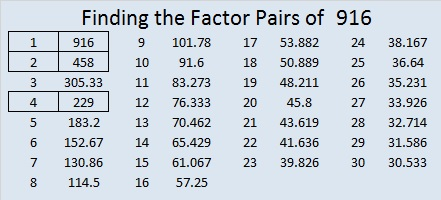# 1687 Fly Me to the Moon!

### Today’s Puzzle:

A witch flying on a broomstick in front of a bright full moon is a common Halloween image. Here is a level 4 puzzle shaped like a broom. If you succeed in solving it, you might just feel like you are flying to the moon, too. Just write the numbers from 1 to 12 in both the first column and in the top row so that those numbers and the given clues form a multiplication table. Best Witches!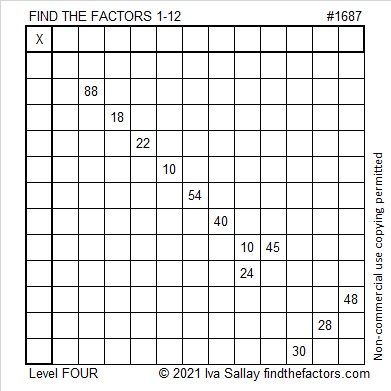### Factors of 1687:

1687 is divisible by 7 because 16 is the double of 8, and the last digit is 7.
217, 427, 637, 847, 1057, 1267, 1477, 1687, 1897 are all divisible by 7.

• 1687 is a composite number.
• Prime factorization: 1687 = 7 × 241.
• 1687 has no exponents greater than 1 in its prime factorization, so √1687 cannot be simplified.
• The exponents in the prime factorization are 1 and 1. Adding one to each exponent and multiplying we get (1 + 1)(1 + 1) = 2 × 2 = 4. Therefore 1687 has exactly 4 factors.
• The factors of 1687 are outlined with their factor pair partners in the graphic below.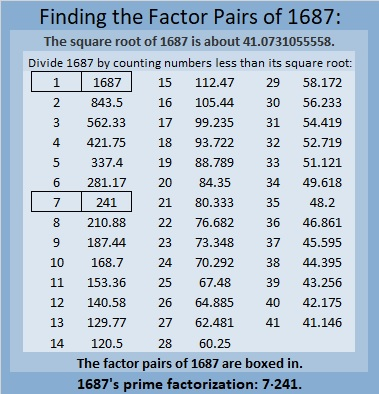### More About the Number 1687:

1687 is the hypotenuse of a Pythagorean triple:
840-1463-1687, which is 7 times (120-209-241).

# 1269 Tangram Witch Puzzle

If you have the seven tangram pieces, then you can create this Halloween witch riding across the moon (or a paper plate). Best Witches creating all kinds of things with those fabulous tiles!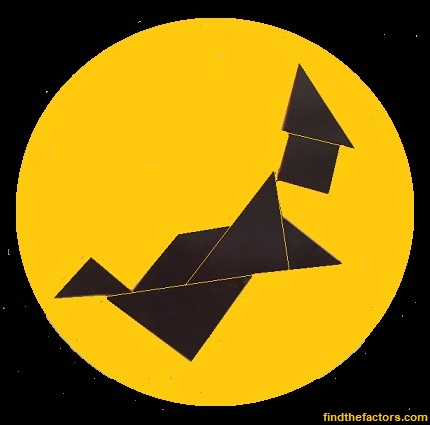In case you would like to know some facts about the number 1269, here’s what I’ve learned:

• 1269 is a composite number.
• Prime factorization: 1269 = 3 × 3 × 3 × 47, which can be written 1269 = 3³ × 47
• The exponents in the prime factorization are 3 and 1. Adding one to each and multiplying we get (3 + 1)(1 + 1) = 4 × 2 = 8. Therefore 1269 has exactly 8 factors.
• Factors of 1269: 1, 3, 9, 27, 47, 141, 423, 1269
• Factor pairs: 1269 = 1 × 1269, 3 × 423, 9 × 141, or 27 × 47
• Taking the factor pair with the largest square number factor, we get √1269 = (√9)(√141) = 3√141 ≈ 35.62303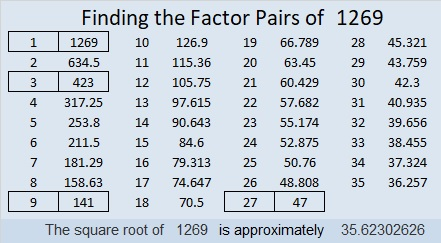1269 is the difference of two squares four different ways:
37² – 10² = 1269
75² – 66² = 1269
213² – 210² = 1269
635² – 634² = 1269

# 916 Witch’s Hat

Today’s puzzle looks a little like a witch’s hat. Solving it could be bewitching. It contains a lot of perfect squares, and it’s only a level 2. Give it a try! Just make sure you don’t write a number greater than ten in the top row or the first column. Use all the numbers from one to ten in both places. There is only one solution.Print the puzzles or type the solution on this excel file: 10-factors-914-922

916 looks the same upside-down as it does right-side up, so it is a Strobogrammatic number.It looks like someone put a spell on that number!

Not only that, in BASE 26, our 916 looks like 196. There is a whole lot of magic going on here!

30² + 4² = 916, so 916 is the hypotenuse of a Pythagorean triple:
240-884-916, which can be calculated from 2(30)(4), 30² – 4², 30² + 4².

• 916 is a composite number.
• Prime factorization: 916 = 2 × 2 × 229, which can be written 916 = 2² × 229
• The exponents in the prime factorization are 2 and 1. Adding one to each and multiplying we get (2 + 1)(1 + 1) = 3 × 2  = 6. Therefore 916 has exactly 6 factors.
• Factors of 916: 1, 2, 4, 229, 458, 916
• Factor pairs: 916 = 1 × 916, 2 × 458, or 4 × 229
• Taking the factor pair with the largest square number factor, we get √916 = (√4)(√229) = 2√229 ≈ 30.2654919